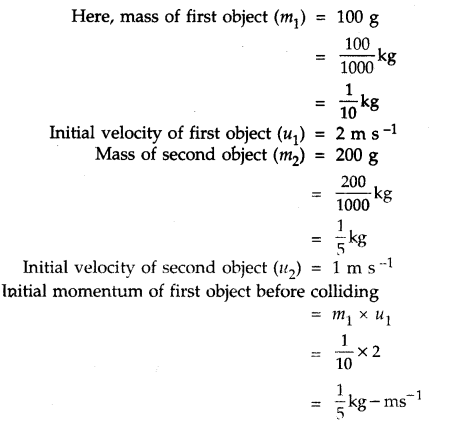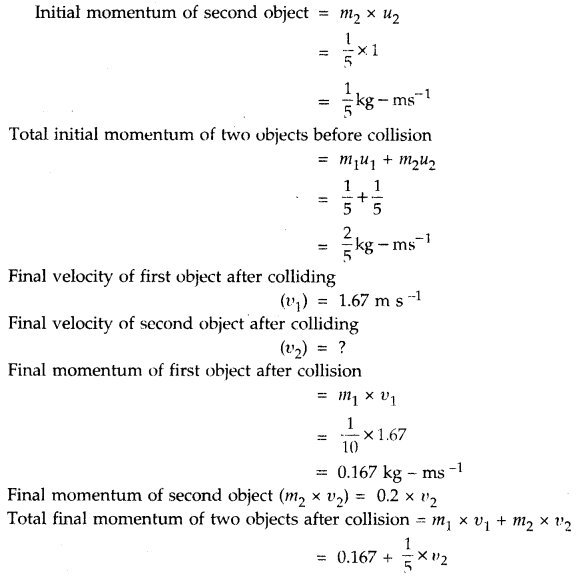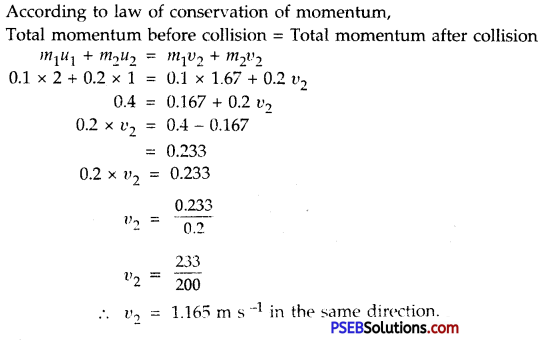# PSEB 9th Class Science Solutions Chapter 9 Force and Laws of Motion

Punjab State Board PSEB 9th Class Science Book Solutions Chapter 9 Force and Laws of Motion Textbook Exercise Questions and Answers.

## PSEB Solutions for Class 9 Science Chapter 9 Force and Laws of Motion

PSEB 9th Class Science Guide Force and Laws of Motion Textbook Questions and Answers

Question 1.
An object experiences a net zero external unbalanced force. Is it possible for the object to be travelling with a non-zero velocity? If yes, state the conditions that must be placed on the magnitude and direction of the velocity. If no, provide a reason.
Yes, it is possible for an object to move with non-zero velocity even when net zero unbalanced force is experienced by it. In this situation the magnitude of velocity and direction will be same. As for example, in case of a rain drop falling freely with constant velocity, the weight of the drop is balanced by upthrust so to say the net unbalanced force on drop is zero.Question 2.
When a carpet is beaten with a stick, the dust comes out of it? Explain.
When a carpet is beaten with a stick, the carpet is set into motion while the dust particles due to inertia tend to remain at rest. In this way dust particles get detached from the carpet and come out of it.

Question 3.
Why is it advised to tie any luggage kept on the roof of a bus with rope?
When a fast-moving bus suddenly takes a turn round a sharp bend then the luggage placed on the roof of a bus gets displaced. The reason for this is that the luggage tends to remain with linear motion while an unbalanced force is applied by the engine to change the direction of the bus so that the luggage kept at the roof of the bus gets displaced. So it is advised to tie the luggage with a rope on the roof of bus.

Question 4.
A batsman hits a cricket ball when then rolls on a level ground. After covering short distance, the ball comes to rest. The ball comes to a stop because
(a) the batsman did not hit the ball hard enough.
(b) velocity is proportional to the force exerted on the ball.
(c) there is a force on the ball opposing the motion.
(d) there is no unbalanced force on the ball so that ball would want to come to rest.
(c) is correct. There is a force of friction on the ball in direction opposite to that of motion.

Question 5.
A truck starts from rest and rolls down a hill with constant acceleration. It travels a distance of 400 m in 20 s. Find its acceleration. Find the force on it if its mass is 7 metric tonnes. (1 tonne = 100 kg)
Solution:
Here initial velocity (u) = 0
Time (t) = 20 s
Distance (s) = 400 m
S = ut + $$\frac{1}{2}$$at2
400 = 0 × 30 + $$\frac{1}{2}$$ × a × (20)2
400 = 0 + $$\frac{1}{2}$$ × a × 20 × 20
400 = $$\frac{1}{2}$$ × 20 × 20 × a
400 = 200 × a
or a = $$\frac{400}{200}$$
∴ a = 2ms-2
Now mass of the truck(m) = 7 tonne
= 7 × 1000 kg
Acceleration (a) = 2ms-2
But Force, F = m × a
= 7000 kg × 2 ms-2
= 14000 kg – ms-2
= 14000 NQuestion 6.
A stone of 1 kg is thrown with a velocity of 20 m s-1 across the frozen surface of a lake and comes to rest after travelling a distance of 50 m. What is the force of friction between the stone and the ice?
Solution:
Here, mass of stone (m) = 1 kg
Initial velocity of stone (u) = 20 ms-1
Distance travelled by the stone (S) = 50 m
Final velocity of stone (υ) = 0 (at rest)
Force of friction between the stone and ice (F) = ?
Using υ2 – u2 = 2aS, we have
(0)2 – (20)2 = 2 × (a) × 50
– 20 × 20 = 100 × a
a = $$\frac{-20 \times 20}{100}$$
a = – 4 ms-2
F = ma = 1 × (- 4)
F = – 4 N
Minus sign shows the force of friction is in direction opposite to direction of motion of stone.

Question 7.
A 8,000 kg engine pulls a train of 5 wagons, each of 2,000 kg along a horizontal track. If the engine exerts a force of 40,000 N and track offers a force of friction of 35,000 N, then calculate the
(a) net accelerating force;
(b) acceleration of the train; and
(c) force of wagon 1 on wagon 2.
Solution:
Mass of the engine = 8000 kg
Mass of 5 wagons = 5 × 2000 kg = 10,000 kg
∴ Total mass of engine and 5 wagons = 8000 kg + 10,000 kg = 18,000 kg
Total force of engine = 40,000 N
Frictional force offered by the track= 5000 N
(a) ∴ Net Accelerating Force (F) = Total force of engine – Frictional force of track
= 40,000 N – 5000 N = 35,000 N

(b) Acceleration of the train (a) = $$\frac{Accelerating force on rail(F)}{Mass of the train(m)}$$
= $$\frac{35000}{18000}$$
= $$\frac{35}{18}$$m-2
= 1.94ms-2

(c) Force exerted by wagon 1 on wagon 2 = Net Accelerating Force – Mass of wagon × Acceleration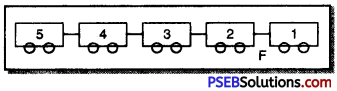= 35000 – 2000 × $$\frac{35}{18}$$
= 35000 – 3888.8
= 31111.2 N

Question 8.
An automobile vehicle has mass of 1,500 kg. What must be the force between vehicle and the road if vehicle is to be stopped with negative acceleration of 1.7 ms-2?
Solution:
Here the mass of automobile (m) = 1500 kg
Acceleration of vehicle (a) = -1.7ms-2
Frictional Force between road and vehicle (F) = ?
We know, F = m x a
= 15000 x (- 1.7)
= – 2550 N
∴ Backward frictional force (F) = 2550 NQuestion 9.
What is the momentum of an object of mass m moving with velocity υ?
(a) (mυ)2; (b) mυ2; (c) $$\frac{1}{2}$$mυ2, (d) mυ.
(d) is correct. Momentum = mυ.

Question 10.
Using a horizontal force of 200 N, we intend to move a wooden cabinet across a floor with constant velocity. What is the friction force that will be exerted on the cabinet?
When no acceleration is to be produced (i.e. body is to be moved with constant velocity), the net force has to be zero. Force of friction should be equal and opposite to the force applied i.e., force of friction has to be 200 N.

Question 11.
Two objects, each of mass 1.5 kg, are moving in the same straight line but in the opposite directions. The velocity of each object is 2.5 ms-1 before the collision during which they stick together. What will be the velocity of combined object after collision?
Solution:
Given, mass of the lirst object (m1) – 1.5 kg
, and mass of the second object (m2) = m1 = 1.5 kg
Initial velocity of the first object (u1) = 2.5 ms-1
Initial velocity of the second object (u2) = – 2.5 ms-1
(Since both the objects move in the direction opposite to each other therefore velocity of first object will be taken as positive and that of the other as negative.)
Suppose after collision the velocity of the combination of two objects is ‘υ’
∴ According to the law of conservation of momentum,
Total momentum before collision = Total momentum after collision
m1u1 + m2u2 = m1υ + m2υ
1.5 × 2.5 + 1.5 × (-2.5) = (1.5 × υ + 1.5 × υ)
1.5 [2.5 + (-2.5)] = (1.5 + 1.5) × υ
1.5 [2.5 – 2.5] = 3 × υ
1.5 × 0 = 3 × υ
0 = 3 × υ
∴ υ = 0 ms-1
i. e. Both the objects will come to rest after collision.

Question 12.
According to the third law of motion when we push on an object, the object pushes back on us with an equal and opposite force. If the object is a massive truck parked along the road side, it will probably not move. A student justifies by answering that the two forces cancel each other. Comment on the logic and explain why the truck does not move.
Student is justified. Friction is equal and opposite to force applied till the force applied crosses the force of limiting friction. When he applies a force slightly more than force of limiting friction, the truck will move. Till the truck moves uniformly, the force applied is exactly equal to force of friction at that instant.Question 13.
A hockey ball of mass 200 g travelling at 10 ms-1 is struck by a hockey stick so as to return it along its original path with a velocity at 5 ms-1. Calculate the change in momentum occurred in the motion of hockey ball by the force applied by hockey stick.
Sol. Mass of the ball (m) = 200 g
= $$\frac{200}{1000}$$kg = 0.2 kg
Initial velocity of the ball (u) = 10 ms-1
Final velocity of the ball (v) = – 5ms-1
[∵ the direction of the ball is opposite to the first direction]
Change in momentum of the ball = Final momentum – Initial momentum.
= mυ – mu
= m (υ – u)
= 0.2 (- 5 – 10)
= 0.2 × (- 15)
= – 3.0 kg – ms-1

Question 14.
A bullet of mass 10 kg travelling horizontally with a velocity of 150 ms-1 strikes a stationary wooden block and come to rest in 0.03 s. Calculate the distance of penetration of the bullet into the block. Calculate the magnitude of force exerted by the wood in block in the bullet.
Solution:
Here, mass of the bullet (m) = 10 g = 0.01 kg
Initial velocity of the bullet (u) = 150 ms-1
Final velocity of the bullet (υ) = 0
Time (t) = 0.03 s
We know, acceleration of the bullet (a) = $$\frac{v-u}{t}$$
= $$\frac{0-150}{0.03}$$
= – 5000 ms-2

(a) Net force exerted by the wooden block on the bullet (F) = m × a
= 0.01 × (- 5000)
= 1 × (-50)
= -50N
∴ Magnitude of force = 50 N

(b) Distance covered by the bullet after penetration in the wooden block (S) = ?
using S = ut + $$\frac{1}{2}$$at2
= 15 × 0.03 + $$\frac{1}{2}$$ × (- 5000) × (0.03)2
= 4.5 + (- 2.25)
= 4.5 – 2.25
S = 2.25 m

Question 15.
An object of mass 1 kg travelling in straight line with a velocity of 10 ms-1 collides with it and sticks to a stationary wooden block of mass 5 kg. Then both move off together in the same straight line. Calculate the total momentum before the impact and just after the impact. Also calculate the velocity of combined object.
Solution:
Mass of the object (m1) = 1 kg
Initial velocity of the object (u1) = 10 ms-1
Mass of the wooden block (m2) = 5 kg
Initial velocity of the wooden block (u2) = 0 [Wooden block at rest]
Suppose ‘υ’ is the velocity of the combination of the object and wooden block after collision
∴ Before collision total momentum of the object and block
= m1u1 + m2u2
= 1 × 10 + 5 × 0
= 10 + 0
= 10kg ms-1 ……………… (i)
After collision. Total momentum of the object and block = m1υ + m2υ
= (m1 + m2) × υ
= (1 + 5) × υ
= 6υ kg m – ms ……… (ii)
According to the law of conservation of momentum,
Total momentum of the combinations before collision = Total momentum of the combination after collision 10 = 6υ
υ = $$\frac{10}{6}$$
∴ υ = $$\frac{5}{3}$$ ms-1
= 1.67 ms-1
Substituting the value of υ in (ii) above
∴ Total momentum of the combination (after collision) = 6υ
= 6 × $$\frac{5}{3}$$
= 10kg – ms-1Question 16.
An object of mass 100 kg is accelerated uniformly from a velocity of 5 ms-1 to 8 ms-1 in 6s. Calculate the initial and final momentum of the object. Also find the force exerted on the object.
Solution:
Here, mass of the object (m) = 100kg
Initial velocity of the object (u) = 5ms-1
Final velocity of the object (v) = 8ms-1
Time interval (t) = 6s
Initial momentum of the object (p1) = m × u
= 100 × 5 = 500 kg – ms-1
Final momentum of the object (p2) = m × υ
= 100 × 8 = 800 kg – ms-1
Force acting on the object (F) = $$\frac{p_{2}-p_{1}}{t}$$
= $$\frac{(800-500) \mathrm{kg}-\mathrm{ms}^{-1}}{6 \mathrm{~s}}$$
= 50kg – ms-2 = 50N

Question 17.
Akhtar, Kiran and Rahul were riding in a motor car that was moving with a high velocity on an express-way when an insect hit the windshield and got struck on wind-screen. Akhtar and Kiran started pondering over the situation. Kiran suggested that the insect suffered a greater change in momentum as compared to the change in momentum of motor car (because change in the velocity of insect was much more than that of motor car). Akhtar said that since the motor car was moving with a larger velocity, it exerted a larger force on the insect. As a result, the insect died. Rahul while putting in entirely new explanation said that both the motor car and the insect experienced the same force and same change in their momentum. Comment on these suggestions.
I agree with Rahul’s explanation. According to law of conservation of momentum, during collision, the momentum of the system (insect and motor car) remains conserved. Therefore, both insect and motor car experience the same force and hence same change in momentum. The insect having smaller mass would suffer greater change in velocity as a result of this, it will crush the insect while the motor car does not suffer any noticeable change in velocity.

Question 18.
How much momentum will a dumbbell of mass 10 kg transfer to the floor if it falls from a height of 80 cm? Take its downward acceleration to be 10 ms-2.
Solution:
Here, momentum of the dumb-bell (m) = 10 kg
Initial velocity of the bell (u) = 0 (at rest)
Distance covered by the bell (S) = (h) = 80 cm
= 0.80 m
Acceleration of the ball (a) = 10 ms-2 (downward direction)
Let υ be the final velocity of the bell on reaching the ground.
Using υ2 – u2 = 2aS
υ2 – (0)2 = 2 × 10 × 0.80
υ2 = 2 × 10 × 0.80
or υ2 = 16
∴ Final velocity of the bell (υ) = $$\sqrt{16}$$ = 4ms
Momentum transferred by the bell to the floor (p) = m × υ
= 10 × 4 = 40 kg – ms-1

Science Guide for Class 9 PSEB Force and Laws of Motion InText Questions and Answers

Question 1.
Which of the following has more inertia:
(a) rubber ball and a stone of the same size?
(b) a bicycle and a train?
(c) a five rupees coin and a one-rupee coin?
We know that mass of an object is the measure of its inertia. The more is the mass of an object, the more is its inertia hence.
(a) a stone of the same size has more inertia than a rubber ball.
(b) a train has more inertia than a bicycle.
(c) a ₹ 5 coin has more inertia than ₹ 1 coin because a ₹ 5 coin has more mass than a ₹ 1 coin.Question 2.
In the following example, try to identify the number of times the velocity of the ball changes:
“A football player kicks a football to another player of his team who kicks the football towards the goal-keeper. The goal-keeper of opposite team collects the football and kicks it towards a player of his own team”. Also identify the agent supplying the force in each case.
To push, to strike or to pull, all these activities act as a force for changing the velocity or for changing the direction of motion of the object. Therefore, in the above given example the velocity of ball changes four times.

1. First time the football player of first-team kicks the football to another player of his team and thus changes the velocity of the football.
2. In second time velocity changes when the second player kicks the football towards the goal-keeper of the opposite team and applies force on the ball.
3. Third time the goal-keeper pushes the ball and reduces its velocity to zero by applying force.
4. The goal-keeper now applies a force by kicking the football towards player of his team. In this case the force increases the velocity of the football.

Question 3.
Explain why some of the leaves may get detached from the tree if we vigorously shake its branch.
Before shaking, the branch of the tree, both the branch and leaves were at rest. When we shake the branch of the tree, branch moves but the leaves remain at rest due to inertia of rest and get detached from the branch.

Question 4.
Why do you fall in the forward direction when a moving bus brakes to a stop and falls backward when it accelerates from rest?
When the bus is moving, whole of our body is moving forward. When brakes are applied, the lower part of our body touching the bus (e.g., feet etc.) comes to rest and upper part of the body not touching the bus continue move forward due to inertia of motion and fall in forward direction.

When the bus suddenly starts and accelerates from rest, the lower part of our body starts moving forward (accelerating) along with the bus while upper part of our body tends to remain at rest due to inertia of rest and we fall backward.

Question 5.
If action is always equal to reaction, explain how a horse can pull a cart?
According to Newton’s third law of motion “Action and Reaction are equal and opposite.”
The horse pulls (Action) the cart with some force in the forward direction and the cart applies equal force on the cart in the backward direction (Reaction). These two forces balance each other. When the horse pushes the ground with its feet in the backward direction with force P along OP it gets reaction R due to ground along OR in the upward direction.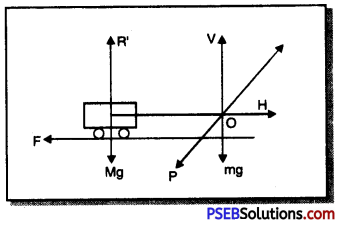This force of reaction can be resolved into two rectangular components.
1. Vertical component ‘V’ which balances the weight mg of the horse and cart in the downward direction.
The horizontal component ‘H’ which helps to move the cart in the forward direction. The force of friction between wheels and ground acts in the backward direction but the horizontal component ‘H’ acts in the forward direction is more than the backward force of friction, it succeeds to move the cart forward.

Question 6.
Explain why is it difficult for a fireman to hold a hose, which ejects large amount of water at a high velocity?
Water is ejected from rubber hose in forward direction with a force (action), it exerts an equal reaction on the hose in backward direction. Due to backward reaction, fire man finds it difficult to hold the hose.Question 7.
From a rifle of mass 4 kg, a bullet df> mass 50 g is fired with an initial velocity of 35 m s-1. Calculate the recoil velocity of the rifle.
Solution:
Mass of the bullet (m1) = 50 g = 0.05 kg
Mass of the rifle (m2) = 4 kg
Initial velocity of the bullet (u1) = 0
Initial velocity of the rifle (u2) = 0
Final velocity of the bullet (υ1) = 35 m s-1
Final velocity of the rifle (υ2) = ?
According to the law of conservation of momentum,
Total initial momentum of bullet and rifle = Total final momentum of the bullet and rifle.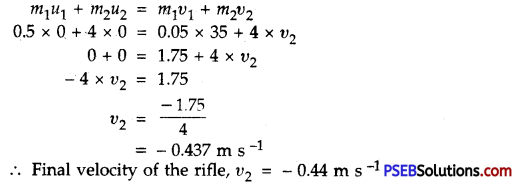∴ Negative sign indicates that the rifle moves in a direction opposite to the direction of motion of the bullet.

Question 8.
Two objects of masses 100 g and 200 g are moving along the same line and direction with velocities of 2 ms-1 and 1ms-1 respectively. They collide and after the collision, the first object moves with a velocity of 1.67 m s-1. Determine the velocity of the second object.
Solution: